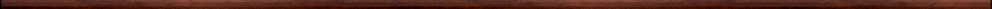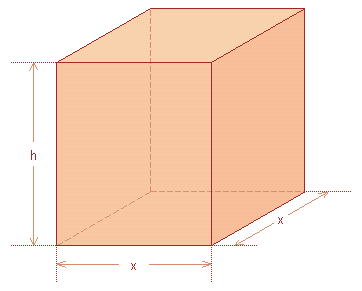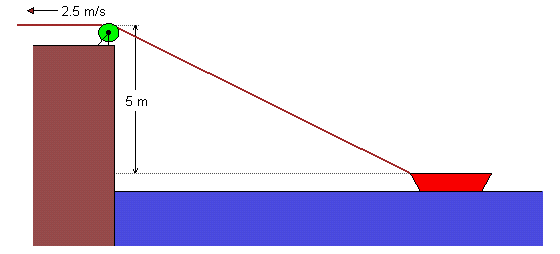Maple worksheets on applications of differentiationBasic calculus topics:

They are all compatible with Classic Worksheet Maple 10.Use of the first and second derivative in curve-sketching - sketch.mws

• The sign of the derivative in connection with curve-sketching.
• The second derivative and curve-sketching.
• Classification of turning points.

Maximum and minimum problems involving area and volume - maxmin1.mws

• Advice for solving max/min problems
• Example 1 - rectangular enclosure fenced on 3 sides
• Example 2 - rectangle under semi-circle
• Example 3 - printed page with margins
• A procedure for drawing a box
• Example 4 - maximizing the volume of an open box
• Example 5 - most economical open box

Miscellaneous max/min problems - maxmin2.mws

• Example 1 - a window with a circular arch
• Example 2 - bending lengths of wire into a square and a circle
• Example 3 - most economical cylindrical can
• Example 4 - shortest travelling time
• Example 5 - pulse rate

Problems involving minimising distance - maxmin3.mws

• A procedure for computing the distance between two points
• The minimum distance from a point to a curve
• Fermat's principle

More max/min problems - maxmin4.mws

• Minimising the curved surface area of a conical tent
• Maximising the volume of a cylinder inscribed in a sphere

Related rates problems - relrates.mws

• Example 1 - an expanding oil slick
• Example 2 - a sawdust pile
• Example 3 - pulling a boatTop of page

Main index